# Test: Chemical Reactions & Equations - 4

## 25 Questions MCQ Test NCERT Textbooks (Class 6 to Class 12) | Test: Chemical Reactions & Equations - 4

Description
Attempt Test: Chemical Reactions & Equations - 4 | 25 questions in 25 minutes | Mock test for UPSC preparation | Free important questions MCQ to study NCERT Textbooks (Class 6 to Class 12) for UPSC Exam | Download free PDF with solutions
QUESTION: 1

### Which of the following product is formed when calcium oxide reacts with water?

Solution:

When calcium oxide (chemical formula: Ca0) reacts with water (chemical formula: H20), the following reaction takes place: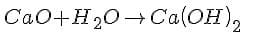The product of this reaction is calcium hydroxide, also known as slaked lime. Thus, when calcium oxide reacts with water, slaked lime is produced.

QUESTION: 2

### The reactions in which more reactive element can displace less reactive element from a compound are called

Solution:

Displacement reaction is a chemical reaction in which a more reactive element displaces a less reactive element from its compound. Both metals and non-metals take part in displacement reactions.

QUESTION: 3

### Decomposition of ferrous sulphate into Fe2O3,SO2 and SO3 occurs in the presence of

Solution:

This is due to the Thermal decomposition reaction in which reactants decomposes to form products.

QUESTION: 4

ZnO+C→Zn+CO is an example of

Solution:

This is an example of a Redox reaction in which both the oxidation and reduction take place simultaneously.

QUESTION: 5

Find the incorrect statement :
(I) oxygen is highly combustible and hydrogen is supporter of combustion,
(II) Oxygen and hydrogen both are highly combustible,
(III) Oxygen and hydrogen both are supporters of combustion,
(IV) Hydrogen is highly combustible and oxygen is supporter of combustion

Solution:

In option (1) , if oxygen is highly combustible then it catch fire. Then how do we live, moreover hydrogen is a combustible substance. In option (2) hydrogen is a combustible substance but not oxygen. In option ( 3) oxygen is the supporter of fire but not the hydrogen.

QUESTION: 6

Which of the following decolourise a blue solution of copper sulphate?

Solution:

Aluminium, zinc and iron are more reactive than copper therefore displaces from its solution.

QUESTION: 7

The chemical formula of lead sulphate is

Solution:

QUESTION: 8

Which one of Fe, Al, Cu, Zn is the least reactive metal?

Solution:
QUESTION: 9

The volume of gas collected in one of the test tubes in electrolysis of water is double than other name the gas

Solution:

Water (H2O) contains two parts hydrogen and one part oxygen. Therefore, the amount of hydrogen and oxygen produced during electrolysis of water is in a 2:1 ratio. During electrolysis, since hydrogen goes to one test tube and oxygen goes to another, the amount of gas collected in one of the test tubes is double of the amount collected in the other.

QUESTION: 10

Milk becomes sour if kept for a long time because of

Solution:

Fats and oils in food kept for a long time get oxidized and become rancid and the taste of food changes and cause infection on eating. This is called rancidity

QUESTION: 11

What happens when dilute HCl is added to iron fillings?

Solution:

Hydrogen gas and iron chloride are produced.
Fe + HCl --> FeCl2 + H2

QUESTION: 12

Why does the colour of copper sulphate solution change when an iron nail is dipped in it?

Solution:

When an iron nail dipped in the copper sulphate solution than iron displaces copper from the copper sulphate because iron is more reactive than copper. Therefore the colour of the copper sulphate solution changes.
The reaction involved is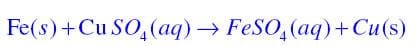QUESTION: 13

What happens when copper metal is added to silver nitrate solution?

Solution:

The reaction is represented as follows:
2AgNO3 + Cu --------> Cu(NO3)2 + 2Ag ↓
Observation: Clear solution of AgNOturns Blue
Type: Single displacement reaction

QUESTION: 14

Fe2O3+2Al→Al2O3+2Fe This reaction is an example of -

Solution:

The given equation is a displacement reaction in which Fe of Fe2O3 has been displaced by Al. Hence, (b) is the correct answer.

QUESTION: 15

Metathesis reactions are called-

Solution:

A double displacement reaction, also known as a double replacement reaction or metathesis, is a type of chemical reaction where two compounds react, and the positive ions (cation) and the negative ions (anion) of the two reactants switch places, forming two new compounds or products.

QUESTION: 16

Solutions of zinc sulphate (I), copper sulphate (II), aluminum sulphate (III) and iron sulphate (IV)

were taken in four test tubes. An iron nail was put in each solution and the system was left undisturbed for an hour. In which set up or set ups the iron nail be coated?

Solution:

In copper sulphate as iron is more reactive than copper.

QUESTION: 17

Chemically rust is

Solution:

Chemically, rust is hydrated ferric oxide (Fe2O3.nH2O)

QUESTION: 18

A student added zinc granules to copper sulphate solution taken in a test tube. Out of the following, the correct observation (s) made by the student will be
I. zinc granules have no regular shape.
II. Zinc granules have silvery grey colour.
III. The colour of zinc granules changed to brownish black.

Solution:

The displacement reaction that occurs is Zn(s)+ CuSO4(aq) → ZnSO4(aq)+Cu(s) (Brownish black)

QUESTION: 19

When a student added zinc granules to dilute HCl, a colour less and odourless gas was evolved, which was tested with a burning match stick it was observed that

Solution:

Explanation: When Zinc is treated with dilute HCl, zinc being more reactive than hydrogen displaces hydrogen from its salt solution and thus produce zinc chloride and hydrogen gas.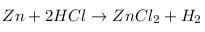This is a single replacement reaction as more reactive zinc displaces hydrogen. the hydrogen gas is odorless and colorless and burns with a pop sound when a burning match stick is brought near it.

QUESTION: 20

Rancidity can be prevented by

Solution:
QUESTION: 21

If the solution of lead nitrate and potassium iodide are mixed together. What is the colour of the precipitate obtained?

Solution:

A precipitation reaction occurs. Yellow precipitates of lead iodide are formed.
The equation for this reaction is Pb(NO3)2 + 2KI -----> PbI2 + 2KNO3.

QUESTION: 22

Match the following with correct response.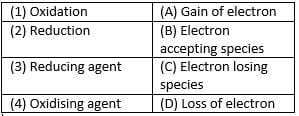Solution:

Oxidation is  loss of electrons. Reduction is gain of electrons. Reducing agents are the electron losing species. Oxidizing agents are electron accepting species.

QUESTION: 23

Match the following with the correct response.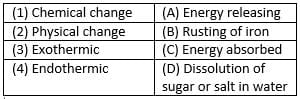Solution:

Rusting of iron is a chemical change. Dissolution of sugar or salt in water is a physical change. In exothermic reaction energy is released. In endothermic reaction energy is absorbed.

QUESTION: 24

Match the following with correct response.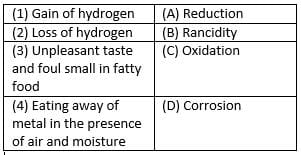Solution:

Reduction is the gain of hydrogen.. Oxidation is the loss of hydrogen.
Rancidity is the unpleasant taste and foul smell in fatty food.
Corrosion is eating away of metal in the presence of air and moisture.

QUESTION: 25

Match the following with correct response.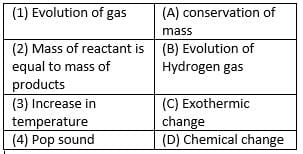Solution:

Evolution of gas is a chemical change. According to the law of conservation of mass , mass of reactant is equal to mass of products. Increase in temperature is exothermic change. Evolution of hydrogen gas causes a pop sound.Use Code STAYHOME200 and get INR 200 additional OFF Use Coupon Code